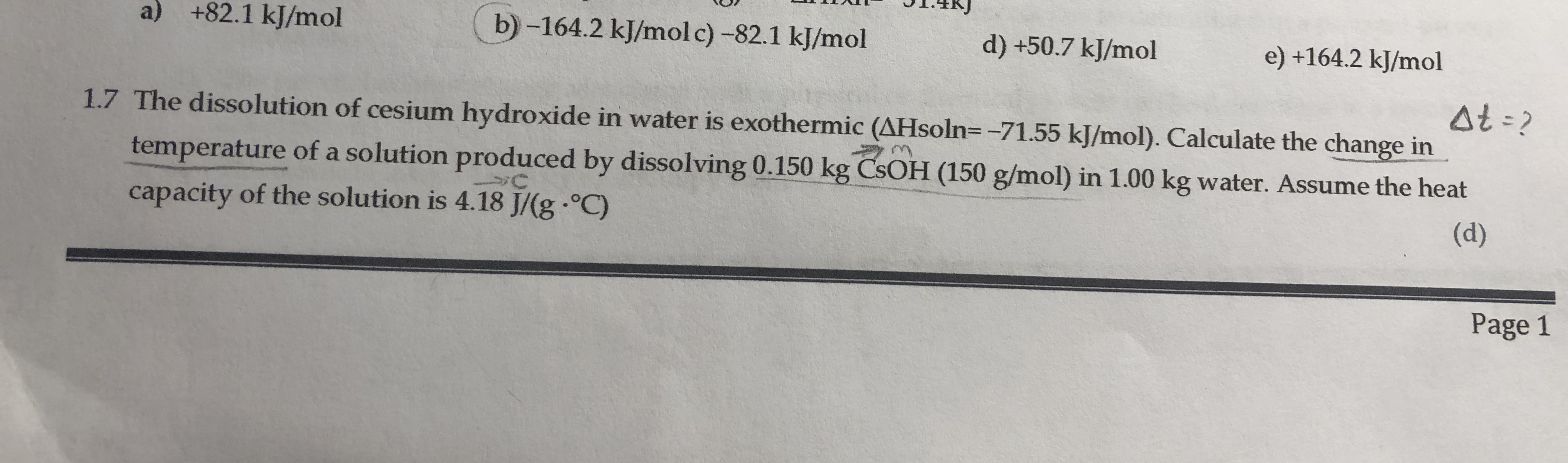# The dissolution of cesium hydroxide in water is exothermic (hsoln = 71.55 kj/mol). Calculate the change in temperature of a solution produced by dissolving 0.150 kg CsOH (150 g/mol) in 1.00 kg water. Assume the heat capacity of the solution 4.18 j/gC